SLOS369G July   2002  – October 2015

PRODUCTION DATA.

1. Features
2. Applications
3. Description
4. Revision History
5. Device Comparison Table
6. Pin Configuration and Functions
7. Specifications
8. Parameter Measurement Information
9. Detailed Description
1. 9.1 Overview
2. 9.2 Functional Block Diagram
3. 9.3 Feature Description
4. 9.4 Device Functional Modes
10. 10Application and Implementation
1. 10.1 Application Information
2. 10.2 Typical Applications
1. 10.2.1 TPA2005D1 with Differential Input
2. 10.2.2 TPA2005D1 with Differential Input and Input Capacitors
1. 10.2.2.1 Design Requirements
2. 10.2.2.2 Detailed Design Procedure
3. 10.2.3 TPA2005D1 with Single-Ended Input
11. 11Power Supply Recommendations
12. 12Layout
1. 12.1 Layout Guidelines
2. 12.2 Layout Examples
13. 13Device and Documentation Support
14. 14Mechanical, Packaging, and Orderable Information

#### Package Options

Refer to the PDF data sheet for device specific package drawings

• DGN|8
• DRB|8
• DRB|8
• DGN|8

## 7 Specifications

### 7.1 Absolute Maximum Ratings

over operating free-air temperature range (unless otherwise noted)(1)
MIN MAX UNIT
VDD Supply voltage(2) In active mode –0.3 6 V
In SHUTDOWN mode –0.3 7 V
VI Input voltage –0.3 VDD + 0.3 V V
TA Operating free-air temperature –40 85 °C
TJ Operating junction temperature –40 85 °C
Tstg Storage temperature –65 150 °C
RL Load resistance 2.5 ≤ VDD ≤ 4.2 V 3.2 (Minimum) Ω
4.2 < VDD ≤ 6 V 6.4 (Minimum) Ω
(1) Stresses beyond those listed under Absolute Maximum Ratings may cause permanent damage to the device. These are stress ratings only, which do not imply functional operation of the device at these or any other conditions beyond those indicated under Recommended Operating Conditions. Exposure to absolute-maximum-rated conditions for extended periods may affect device reliability.
(2) For the MSOP (DGN) package option, the maximum VDD should be limited to 5 V if short-circuit protection is desired.

### 7.2 ESD Ratings

VALUE UNIT
V(ESD) Electrostatic discharge Human-body model (HBM), per ANSI/ESDA/JEDEC JS-001(1) ±3000 V
Charged-device model (CDM), per JEDEC specification JESD22-C101(2) ±1500
(1) JEDEC document JEP155 states that 500-V HBM allows safe manufacturing with a standard ESD control process.
(2) JEDEC document JEP157 states that 250-V CDM allows safe manufacturing with a standard ESD control process.

### 7.3 Recommended Operating Conditions

over operating free-air temperature range (unless otherwise noted)
MIN NOM MAX UNIT
VDD Supply voltage 2.5 5.5 V
VIH High-level input voltage SHUTDOWN 2 VDD V
VIL Low-level input voltage SHUTDOWN 0 0.8 V
RI Input resistor Gain ≤ 20 V/V (26 dB) 15
VIC Common mode input voltage range VDD = 2.5 V, 5.5 V, CMRR ≤ –49 dB 0.5 VDD-0.8 V
TA Operating free-air temperature –40 85 °C

### 7.4 Thermal Information

THERMAL METRIC(1) TPA2005D1 UNIT
ZQY (MicroStar Junior) GQY (MicroStar Junior) DRB (VSON) DGN (MSOP PowerPAD)
15 PINS 15 PINS 8 PINS 8 PINS
RθJA Junction-to-ambient thermal resistance 92.7 92.7 50.9 57.2 °C/W
RθJC(top) Junction-to-case (top) thermal resistance 120.5 120.5 66.2 53.8 °C/W
RθJB Junction-to-board thermal resistance 104 104 25.9 33.7 °C/W
ψJT Junction-to-top characterization parameter 3.1 3.1 1.4 1.9 °C/W
ψJB Junction-to-board characterization parameter 44.8 44.8 26 33.47 °C/W
RθJC(bot) Junction-to-case (bottom) thermal resistance n/a n/a 7 6.4 °C/W

### 7.5 Electrical Characteristics

TA = 25°C, over operating free-air temperature range (unless otherwise noted)
PARAMETER TEST CONDITIONS MIN TYP MAX UNIT
|VOS| Output offset voltage (measured differentially) VI = 0 V, AV = 2 V/V, VDD = 2.5 V to 5.5 V 25 mV
PSRR Power supply rejection ratio VDD = 2.5 V to 5.5 V –75 –55 dB
CMRR Common mode rejection ratio VDD = 2.5 V to 5.5 V, VIC= VDD/2 to 0.5 V,
VIC= VDD/2 to VDD- 0.8 V
–68 –49 dB
|IIH| High-level input current VDD = 5.5 V, VI = 5.8 V 50 μA
|IIL| Low-level input current VDD = 5.5 V, VI = 0.3 V 1 μA
I(Q) Quiescent current VDD = 5.5 V, no load 3.4 4.5 mA
VDD = 3.6 V, no load 2.8
VDD = 2.5 V, no load 2.2 3.2
I(SD) Shutdown current V (SHUTDOWN) = 0.8 V, VDD = 2.5 V to 5.5 V 0.5 2 μA
rDS(on) Static drain-source on-state resistance VDD = 2.5 V 770
VDD = 3.6 V 590
VDD = 5.5 V 500
Output impedance in SHUTDOWN V (SHUTDOWN) = 0.8 V >1
f(sw) Switching frequency VDD = 2.5 V to 5.5 V 200 250 300 kHz
Gain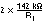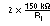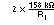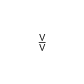### 7.6 Operating Characteristics

TA = 25°C, Gain = 2 V/V, RL = 8 Ω (unless otherwise noted)
PARAMETER TEST CONDITIONS MIN TYP MAX UNIT
PO Output power THD + N= 1%, f = 1 kHz, RL = 8 Ω VDD = 5 V 1.18 W
VDD = 3.6 V 0.58
VDD = 2.5 V 0.26
THD + N= 10%, f = 1 kHz, RL = 8 Ω VDD = 5 V 1.45 W
VDD = 3.6 V 0.75
VDD = 2.5 V 0.35
THD+N Total harmonic distortion plus noise PO = 1 W, f = 1 kHz, RL = 8 Ω VDD = 5 V 0.18%
PO = 0.5 W, f = 1 kHz, RL = 8 Ω VDD = 3.6 V 0.19%
PO = 200 mW, f = 1 kHz, RL = 8 Ω VDD = 2.5 V 0.20%
kSVR Supply ripple rejection ratio f = 217 Hz, V(RIPPLE) = 200 mVpp
Inputs ac-grounded with Ci = 2 μF
VDD = 3.6 V –71 dB
SNR Signal-to-noise ratio PO= 1 W, RL = 8 Ω VDD = 5 V 97 dB
Vn Output voltage noise VDD = 3.6 V, f = 20 Hz to 20 kHz,
Inputs ac-grounded with Ci = 2 μF
No weighting 48 μVRMS
A weighting 36
CMRR Common mode rejection ratio VIC = 1 Vpp , f = 217 Hz VDD = 3.6 V –63 dB
ZI Input impedance 142 150 158
Start-up time from shutdown VDD = 3.6 V 9 ms

### 7.7 Typical Characteristics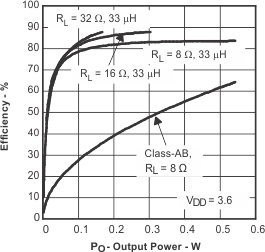Figure 1. Efficiency vs Output Power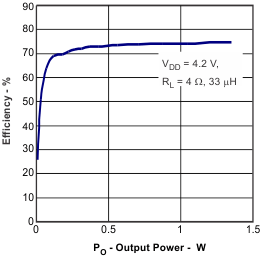Figure 3. Efficiency vs Output Power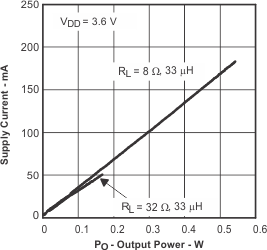Figure 5. Supply Current vs Output Power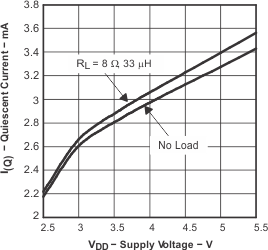Figure 7. Quiescent Current vs Supply VoltageFigure 9. Output Power vs Supply VoltageFigure 11. Output Power vs Load Resistance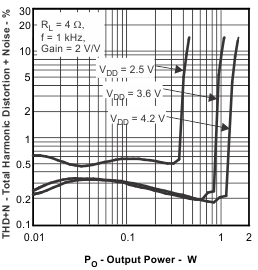Figure 13. Total Harmonic Distortion + Noise vs Output Power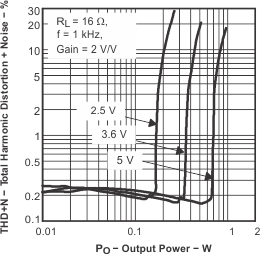Figure 15. Total Harmonic Distortion + Noise vs Output Power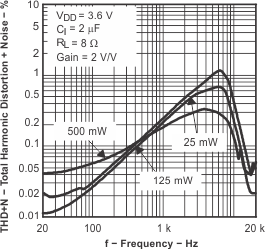Figure 17. Total Harmonic Distortion + Noise vs Frequency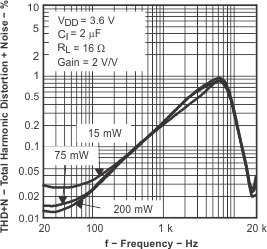Figure 19. Total Harmonic Distortion + Noise vs FrequencyFigure 21. Total Harmonic Distortion + Noise vs Frequency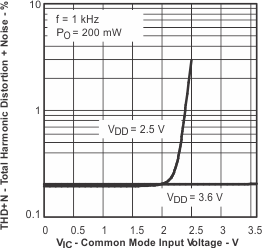Figure 23. Total Harmonic Distortion + Noise vs
Common Mode Input VoltageFigure 25. Supply Voltage Rejection Ratio vs Frequency 25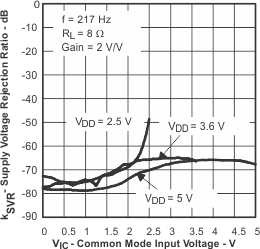Figure 27. Supply Voltage Rejection Ratio vs
Common-mode Input Voltage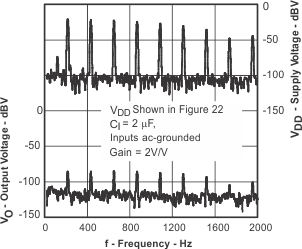Figure 29. GSM Power Supply Rejection vs Frequency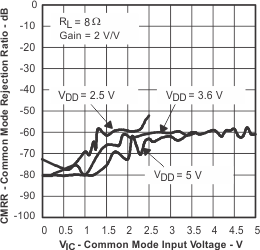Figure 31. Common-mode Rejection Ratio vs
Common-mode Input Voltage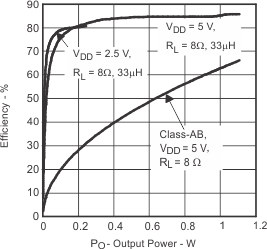Figure 2. Efficiency vs Output Power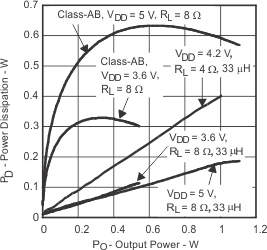Figure 4. Power Dissipation vs Output Power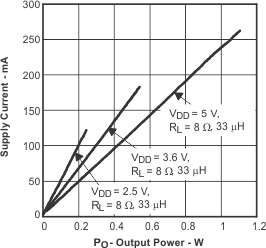Figure 6. Supply Current vs Output Power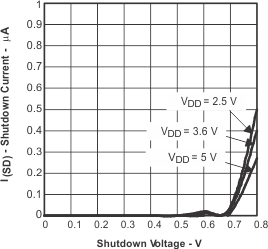Figure 8. Shutdown Current vs Shutdown VoltageFigure 10. Output Power vs Supply Voltage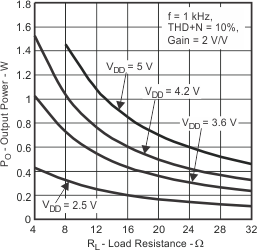Figure 12. Output Power vs Load Resistance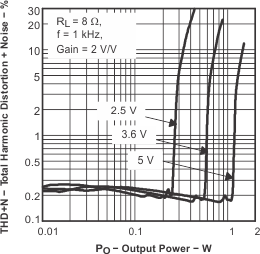Figure 14. Total Harmonic Distortion + Noise vs Output Power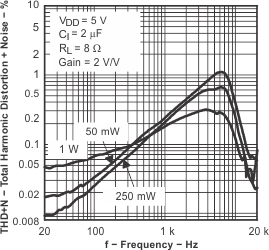Figure 16. Total Harmonic Distortion + Noise vs Frequency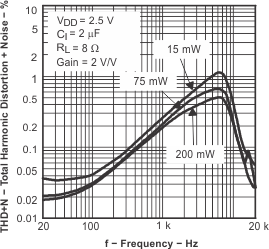Figure 18. Total Harmonic Distortion + Noise vs Frequency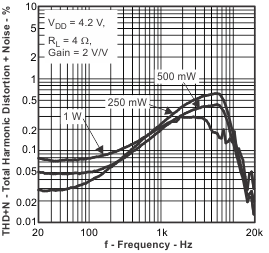Figure 20. Total Harmonic Distortion + Noise vs Frequency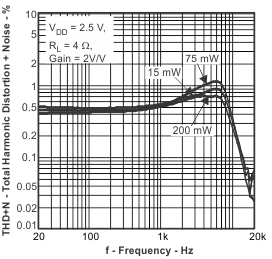Figure 22. Total Harmonic Distortion + Noise vs Frequency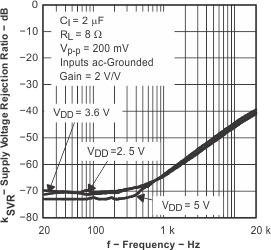Figure 24. Supply Voltage Rejection Ratio vs Frequency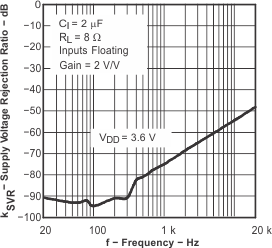Figure 26. Supply Voltage Rejection Ratio vs FrequencyFigure 28. GSM Power Supply Rejection vs Time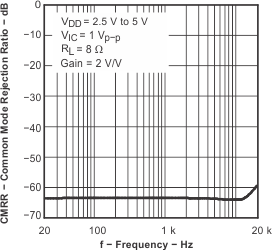Figure 30. Common-mode Rejection Ratio vs Frequency